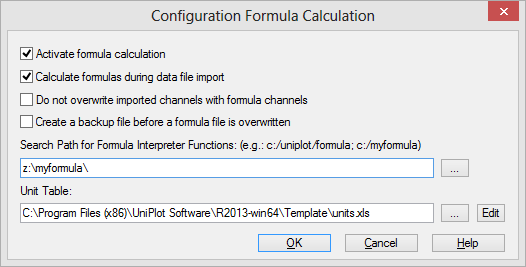# Tools=>Formula Configuration¶

Some settings for to control the calculation or formula channels can be set in this dialog box. See Formula Interpreter 1 - Introduction.Activate Formula Calculation
If this option is set the formula calculator is active. Example: If you press F9 in the data browser formulas will be calculated. If you edit an NC file formulas will be recalculated when the browser is closed.
Calculate Formulas during Import
If this option is set formulas are calculated during the import process.
Do not overwrite imported channels with formula channels
If this option is set only formulas are calculated which are not already present in the data file.
Search Path for Formula Interpreter Functions
The search path field can contain more than one path. UniPlot will load all formula files `.ic` found in each specified path. Separate paths with a semi-colon. New formulas will be added to the last specified directory.
Create a backup file before a formula file is overwritten
If this option is set, a copy of a formula file with the extension .bak is saved in the same directory before the formula file is modified.
Units Table
To select a unit table click on the `...` button. The default table is an Excel file in the installation directory of UniPlot. The file name is `template\units.xlsx`. See also units.

id-692240